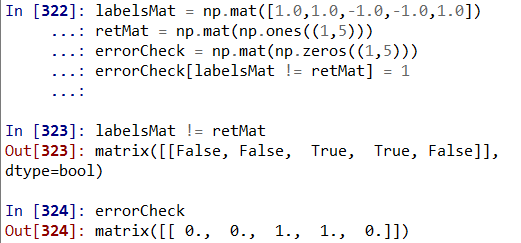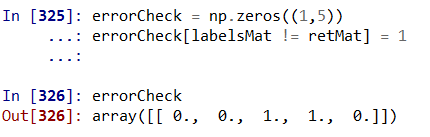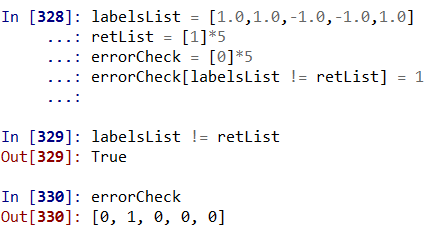• 本文实例讲述了python+numpy实现的基本矩阵操作。分享给大家供大家参考，具体如下：#! usr/bin/env python# coding: utf-8# 学习numpy中矩阵的代码笔记# 2018年05月29日15:43:40# 参考网站：...

本文实例讲述了python+numpy实现的基本矩阵操作。分享给大家供大家参考，具体如下：
#! usr/bin/env python
# coding: utf-8
# 学习numpy中矩阵的代码笔记
# 2018年05月29日15:43:40
# 参考网站：http://cs231n.github.io/python-numpy-tutorial/
import numpy as np
#==================矩阵的创建，增删查改，索引，运算=================================#
#==================矩阵的创建，增删查改=================================#
# # 创建行向量
# x = np.array([1,2,3])
# # 修改某个值
# x = 0
# 注意下标索引从0开始，与MATLAB不一样
# print(x)
# print(x.shape)
# print(type(x))
#
# # 创建二维与多维矩阵
# matrix = np.array([[1,2,3],[1,2,3],[2,3,4]]) # 注意这里有一个小括号，小括号中还有一个中括号
# # 取出某个元素
# a1 = matrix
# print(a1)
# print(matrix.shape)
#
# # # 创建特殊矩阵
# # 0矩阵
# zeros = np.zeros((2,2))# 注意，这里有两个小括号，并且返回浮点型数据，而不是整形
# print(zeros)
#
# # 创建1矩阵
# ones = np.ones([3,3])# 注意这里也是两个括号，其中里面的小括号也可是中括号，但是不建议使用
# print(ones)
#
# # 创建元素相同()的矩阵
# full = np.full((2,3),2) #其中第一个括号表示矩阵大小，后面的数字表示填充的数字
# print(full)
#
# # 创建对角数为1的矩阵
# diag = np.eye(3,3)#注意这里如果行列数不同，只会让行列下标相等的元素为1
# print(diag)
#
# # 创建随机矩阵(值在0到1之间)，注意这个方式不可以重复，也就是随机不可以全部重现，每次运行都会不一样
# random = np.random.random((2,3))
# 写到这里，我需要说明一点，就是如何确定括号的个数
# numpy下的方法肯定是有一个小括号的，且不可以改变
# 想要表达多维阵列，则需要输入一个元祖(小括号)或者列表(中括号)来创建，这时就需要小括号或者中括号
# 如果是自己手敲出多维阵列，每一行需要中括号表示，用逗号分离每一行，然后外层再用一个中括号表示整个矩阵，然后再作为一个举证输入函数中
# print(random)
#=======================矩阵的索引，切片=========================#
metaMatrix = np.array([[1,2,3,4],[5,6,7,8],[9,10,11,12]])#用逗号，而不能用空格
# # 单个元素的索引
# a = metaMatrix
# b = metaMatrix[0,0] # 这里不能使用小括号
# print(a)
# print(b)
#
# # 切片操作
# a_ = metaMatrix[0:2,1]# 注意这里冒号后面的数是不索引的，如果缺省就是到最后，冒号前是可以得到的
# # 冒号后的数不索引，这时python的特点，与MATLAB不一样
# print(a_)
#
# # 注意切片操作后矩阵维度的变化
# a1 = metaMatrix[0:1,:]
# a2 = metaMatrix[0,:]
# b = metaMatrix[0,1]
# print(a1)
# print(a2)
# print(b)
# # 注意到这两行代码得到的数据是一样的，但是维度已经发生了变化
# print(a1.shape) #a1仍然是矩阵
# print(a2.shape) #a2则是一个行向量，相比原矩阵，这里就少了一个维度，这与MATLAB有点不同
# print(b.shape) #b是没有维度的，就是一个数而已
#
# # 利用已有矩阵创建新矩阵，方法比较多样化
# SrcMatrix = np.array([[1,2], [3, 4], [5, 6]])
# print(SrcMatrix)
# # 利用矩阵的方式索引原有矩阵
# matrix1 = SrcMatrix[[0,1],[1,1]]# 这时将两个中括号的对应元素组合起来进行索引，是单个元素索引的扩展
# # 进行单个元素索引，然后组合起来，并用np.array创建成np的数组
# matrix2 = np.array([SrcMatrix,SrcMatrix])
# # 如果不用np.array来创建成np的矩阵，就会导致数据格式的变化,对应的操作就会发生变化
# matrix3 = [SrcMatrix,SrcMatrix]
# print(matrix1)
# print(matrix2)
# print(matrix3)
# print(type(matrix1))
# print(type(matrix2))
# print(type(matrix3))
#
# # numpy矩阵的元素索引方式可以用于改变或者选择矩阵不同行的元素(不仅仅是同一列的数据)
# a = np.array([[1,2,3], [4,5,6], [7,8,9], [10, 11, 12]])
# b = np.array([0,2,0,1])
# # 先介绍一下np.arrange()函数，表示创建一个从起始值到结束值少1(前面提到过，python中经常不到这个值)的行向量，也可以设定步长
# c = a[np.arange(4),b] #其实就是相当于矩阵方式索引一个矩阵中的元素(这比MATLAB中更加自由一些)
# print(c)
# # 改变矩阵的指定元素
# a[np.arange(4),b] += 10
# print(a)
#
# # 布尔型阵列，可以用来索引一些满足特定条件的元素
# matrix = np.array([[1,2],[3,4],[5,6]])
# bool_id = matrix>2 # 也可以写成bool_id =(matrix>2),注意，写成中括号就是不同含义了
# print(bool_id)
# print(matrix[bool_id])
# # 可以将上面两行代码合成一行
# matrix_ = matrix[matrix>2]# 注意，这里得到的是一维向量
# print(matrix_)
#
#=========================numpy array的数据类型=======================================#
# # numpy的array的数据类型是自动识别的，但也可以指定
# # 如果输入为整形，则会给数据的类型定义为int64
# matrix1 = np.array([1,2,3])
# print(matrix1.dtype)
# # 如果输入的数据为小数点，则会给数据类型定义为float64
# matrix2 = np.array([1.0,2.0,3.0])
# print(matrix2.dtype)
# # 如果有浮点型也有整形数据，会赋值给占字节数多的数据类型,且对应为64的
# matrix3 = np.array([1,2.0])
# print(matrix3.dtype)
# # 也可以指定数据类型
# matrix4 = np.array([1,2],dtype=np.int8)
# print(matrix4.dtype)
# # 当数据本身和指定的数据类型不符合时,会将数据转化成指定的数据类型，有可能会发生溢出
# matrix5 = np.array([1,2000000,3.1],dtype=np.int8)
# print(matrix5)
# print(matrix5.dtype)
#=========================矩阵的运算===================================#
#
# # 两种加法和减法，乘除
# x = np.array([[1,2],[3,4]])
# y = np.array([[5,6],[7,8]])
# sum1 = x + y# 直接使用加法
# print(sum1)
# print(sum2)
#
# substract1 = x - y
# substract2 = np.subtract(x,y)
# print(substract1)
# print(substract2)
#
# prodution1 = x * y# 这是对应元素的乘法
# prodution2 = np.multiply(x,y)
# print(prodution1)
# print(prodution2)
#
# devide1 = x/y
# devide2 = np.divide(x,y)
# # 注意矩阵进行运算时，数据类型不改变，因此，需要注意溢出现象等
# print(devide1)
# print(devide2)
#
# # 矩阵的两种向量乘法(使用dot)
# x = np.array([[1,2],[3,4]])
# y = np.array([[5,6],[7,8]])
# multiDot1 = x.dot(y)
# multiDot2 = np.dot(x,y)
# print(multiDot1)
# print(multiDot2)
#
# # 矩阵运算基本函数
# x = np.array([[1,2],[3,4]])
# # 求和函数
# # 对所有元素求和
# sum_all = np.sum(x)
# # 对列求和
# sum_column = np.sum(x, 0)# 注意和MATLAB中的区分一下。
# # 对行求和
# sum_row = np.sum(x, 1)
# print(sum_all)
# print(sum_column)
# print(sum_row)
#
# # 矩阵的转置
# x = np.array([[1,2],[3,4]])
# transform = x.T
# print(transform)
#
# # 考虑将一个常量行向量加到一个矩阵的每一行上
# # 下面会将x行向量加到y矩阵的每一行上(但是这个方法由于有显示循环，而显示循环比较慢一些，我们经常会采用其他方法)
# y = np.array([[1,2,3], [4,5,6], [7,8,9], [10, 11, 12]])
# x = np.array([1, 0, 1])
# y_ = np.empty_like(y) # 创建一个和y相同维度的矩阵，但没有放内容，但是已经开辟了一块内存，其中的数据可能随机
# print(y_)
# for i in range(4):
# y_[i,:] = y[i,:] + x
# print(y_)
# # 另一种方法是我们先将x复制3份，垂直放置，组成一个矩阵，再进行矩阵加法
# x_ = np.tile(x,(4,1))# np.tile表示复制,(4,1)表示将x作为元素，组成4*1的矩阵形式
# print(y__)
# # 实际上，如果不对x进行处理，而直接将两者相加，如果x和y满足一些条件，x会自动复制
# # 条件是x和y在一个维度上相等，另一个维度上不一样并且可以通过复制可以实现维度相等，则会自动复制
# print(y+x)
# # 这里进行一个其他的测试
# print(x.T+y.T)# 可以看出可以实现列的复制
# 这里进行都不为向量的相加
# a1 = np.array([[1,2],[3,4],[5,6],[7,8]])
# a2 = np.array([[1,0],[0,1]])
# print(a1+a2)# 这里会出错，说明只能自动进行一维数据的复制，多维数据不支持自动复制，而需要显式复制
# # 同样的，加法，减法和除法也都适合上面的自动复制原理
# 将一个矩阵或者向量进行维度的调整
x1 = np.array([1,2,3])
y1 = np.array([1,2])
# 实现x1和y1转置的矩阵乘法，可以先将y1变成列向量
print(np.multiply(x1, np.reshape(y1,(2,1))))
# 试一下其他的维度变化
x2 = np.array([[1,2],[3,4],[5,6],[7,8]])
print(np.reshape(x2, (2,4)))
print(np.reshape(x2, (4,2)))# 基本上按照西安航后列的顺序进行
更多关于Python相关内容感兴趣的读者可查看本站专题：《Python数学运算技巧总结》、《Python数据结构与算法教程》、《Python函数使用技巧总结》、《Python字符串操作技巧汇总》及《Python入门与进阶经典教程》
希望本文所述对大家Python程序设计有所帮助。
本文标题: python+numpy实现的基本矩阵操作示例
本文地址: http://www.cppcns.com/jiaoben/python/266004.html

展开全文• What's a faster way to do this operation on python using numpy and scipy. m,n = R.shape for i in range(m): for j in range(n): R[i,j] *= (1 if R[min(0,i-1):max(m, i+2), min(0,j-1):max(n,j+2)].max() ==...Given: R is an mxn float matrix
Output: O is an mxn matrix where O[i,j] = R[i,j] if (i,j) is a local max and O[i,j] = 0 otherwise. Local maximum is defined as the maximum element in a 3x3 block centered at i,j.
What's a faster way to do this operation on python using numpy and scipy.
m,n = R.shape
for i in range(m):
for j in range(n):
R[i,j] *= (1 if R[min(0,i-1):max(m, i+2), min(0,j-1):max(n,j+2)].max() == R[i,j] else 0)
解决方案In : from scipy.ndimage import maximum_filter
Here's a sample R:
In : R
Out:
array([[3, 3, 0, 0, 3],
[0, 0, 2, 1, 3],
[0, 1, 1, 1, 2],
[3, 2, 1, 2, 0],
[2, 2, 1, 2, 1]])
Get the maximum on 3x3 windows:
In : mx = maximum_filter(R, size=3)
In : mx
Out:
array([[3, 3, 3, 3, 3],
[3, 3, 3, 3, 3],
[3, 3, 2, 3, 3],
[3, 3, 2, 2, 2],
[3, 3, 2, 2, 2]])
Compare mx to R; this is a boolean matrix:
In : mx == R
Out:
array([[ True, True, False, False, True],
[False, False, False, False, True],
[False, False, False, False, False],
[ True, False, False, True, False],
[False, False, False, True, False]], dtype=bool)
Use np.where to create O:
In : O = np.where(mx == R, R, 0)
In : O
Out:
array([[3, 3, 0, 0, 3],
[0, 0, 0, 0, 3],
[0, 0, 0, 0, 0],
[3, 0, 0, 2, 0],
[0, 0, 0, 2, 0]])

展开全文• python 判断boolPython bool() function returns Boolean value for an object. The bool class has only two instances – True and False. This class can’t be extended. Python bool（）函数返回对象的布尔值。...

bool型数组python

Python bool() function returns Boolean value for an object. The bool class has only two instances – True and False. This class can’t be extended.
Python bool（）函数返回对象的布尔值。 bool类只有两个实例– True和False。 此类无法扩展。
Python bool（） (Python bool())
Python bool() function uses standard truth testing rules to convert the specified argument object to Boolean value.
Python bool（）函数使用标准的真值测试规则将指定的参数对象转换为布尔值。

Some of the rules used to return Boolean value are:
用于返回布尔值的一些规则是：
Any object Boolean value is considered true if it’s not implementing __bool__() function and __len__() functions. 如果未实现__bool __（）函数和__len __（）函数，则任何对象布尔值都被视为true。 If the object doesn’t define __bool__() function but defines __len__() function, then __len__() function is used to get the boolean value of object. If __len__() returns 0, then bool() function will return False otherwise True. 如果对象没有定义__bool __（）函数而是定义了__len __（）函数，则使用__len __（）函数获取对象的布尔值。 如果__len __（）返回0，则bool（）函数将返回False，否则返回True。 Boolean value will be False for None and False constants. 对于None和False常量，布尔值将为False 。 Boolean value will be False for zero value numbers such as 0, 0.0, 0j, Decimal(0), and Fraction(0, 1). 对于零值数字（例如0、0.0、0j，Decimal（0）和Fraction（0，1）），布尔值将为False。 Boolean value will be False for empty sequences (tuple, dict) and collections, such as ”, (), [], {} etc. 对于空序列（ tuple ， dict ）和集合 （例如”，（），[]，{}等），布尔值将为False。
Python bool（）示例 (Python bool() example)
Let’s look at some simple examples of bool() with bool instances and None.
让我们看一下带有bool实例和None的bool（）的一些简单示例。
x = True
b = bool(x)

print(type(x))  # <class 'bool'>
print(type(b))  # <class 'bool'>
print(b)  # True

x = False
b = bool(x)
print(b)  # False

x = None
b = bool(x)

print(type(x))  # <class 'NoneType'>
print(type(b))  # <class 'bool'>
print(b)  # False
The output is self-explained and provided in the comments.
输出是不言自明的，并在注释中提供。
带有字符串的Python bool（） (Python bool() with strings)
# string examples
x = 'True'
b = bool(x)

print(type(x))  # <class 'str'>
print(type(b))  # <class 'bool'>
print(b)  # True

x = 'False'
b = bool(x)
print(b)  # True because len() is used

x = ''
print(bool(x))  # False, len() returns 0
带有数字的Python bool（） (Python bool() with numbers)
from fractions import Fraction
from decimal import Decimal

print(bool(10))  # True
print(bool(10.55))  # True
print(bool(0xF))  # True
print(bool(10 - 4j))  # True

print(bool(0))  # False
print(bool(0.0))  # False
print(bool(0j))  # False
print(bool(Decimal(0)))  # False
print(bool(Fraction(0, 2)))  # False
具有集合和序列的Python bool（）函数 (Python bool() function with collections and sequences)
tuple1 = ()
dict1 = {}
list1 = []
print(bool(tuple1))  # False
print(bool(dict1))  # False
print(bool(list1))  # False
带有自定义对象的Python bool（）函数 (Python bool() function with custom object)
Let’s see what happens with custom object. I will not define __bool__() and __len__() functions for the object.
让我们看看自定义对象会发生什么。 我不会为该对象定义__bool __（）和__len __（）函数。

class Data:
id = 0

def __init__(self, i):
self.id = i

d = Data(0)
print(bool(d))
d = Data(10)
print(bool(d))
Output:
输出：
True
True
Since none of __bool__() and __len__() functions are defined, object boolean value is returned as True.
由于未定义__bool __（）和__len __（）函数，因此对象布尔值将返回True。

Let’s add __len__() function to the Data class.
让我们向Data类添加__len __（）函数。
# returns 0 for id <= 0, else id
def __len__(self):
print('len function called')
if self.id > 0:
return self.id
else:
return 0
Output:
输出：
len function called
False
len function called
True
It’s clear that __len__() function is called by bool(). When 0 is returned, bool() function is returning False. When positive integer is returned, then bool() function is returning True.
显然__len __（）函数是通过bool（）调用的。 当返回0时，bool（）函数将返回False。 当返回正整数时，则bool（）函数将返回True。
Now let’s add __bool__() function to Data class:
现在，将__bool __（）函数添加到Data类：
# returns True for id > 0 else False
def __bool__(self):
print('bool function called')
return self.id > 0
Now the above snippet output will be:
现在上面的代码片段输出将是：
bool function called
False
bool function called
True
It’s clear from the output that if both __bool__() and __len__() functions are defined for the object, then __bool__() function is used to get the Boolean value of object.
从输出中很明显，如果同时为对象定义了__bool __（）和__len __（）函数，则可以使用__bool __（）函数获取对象的布尔值。

GitHub Repository.
GitHub存储库中检出完整的python脚本和更多Python示例。

Reference: Official Documentation
参考： 官方文档

翻译自: https://www.journaldev.com/22669/python-bool

bool型数组python

展开全文java javascript php c++
• 1. 先来看numpy矩阵，labelsMat ! = retMat语句会对两个矩阵中的每个元素进行比较，返回的boolean型矩阵形状和原矩阵一致。...3. Python列表则有所不同，labelsList != retList会直接比较整个列表是否一样，如
1. 先来看numpy矩阵，labelsMat ! = retMat语句会对两个矩阵中的每个元素进行比较，返回的boolean型矩阵形状和原矩阵一致。将其作为索引，errorCheck会在
为True的位置操作。2. numpy 数组和矩阵没什么区别，可以完成类似操作3. Python列表则有所不同，labelsList != retList会直接比较整个列表是否一样，如果不是，则返回True,是在返回False。
将该boolean值作为列表的索引时，True会被认为是位置1，False是位置0.展开全文numpy boolean 索引 列表
• 目录 合并数据集--数据库风格的DataFrame合并 索引上的合并 轴向连接 合并重叠数据 重塑和轴向旋转--重塑层次化索引 将长格式转换为宽格式 数据转换--移除重复数据 ...pandas中矢量化的字符串函数...
• 这篇文章主要介绍了Python创建对称矩阵的方法,结合实例形式分析了Python基于numpy模块实现矩阵运算的相关操作技巧,需要的朋友可以参考下本文实例讲述了Python创建对称矩阵的方法。分享给大家供大家参考，具体如下：...
• 本文实例讲述了Python创建对称矩阵的方法。分享给大家供大家参考，具体如下：对称（实对称）矩阵也即：step 1：创建一个方阵>>> import numpy as np>>> X = np.random.rand(5**2).reshape(5, 5)>>> Xarray([[ 0....
• 1.矩阵的初始化 import numpy as np（1）.创建全X的矩阵 myzero = np.zeros([3,5]) #全0的矩阵 （2）随机矩阵 myrand = np.random.rand(3,4) (3) 单位阵 myeye = np.eye(3) (4)矩阵运算 from numpy import * 加减乘...numpy
• ## python scipy 稀疏矩阵详解

万次阅读 多人点赞 2019-08-25 16:40:38
文章目录稀疏矩阵格式coo_matrixcsr_matrixcsc_matrixlil_matrixdok_matrixdia_matrixbsr_matrix实用函数经验总结 稀疏矩阵格式 coo_matrix   coo_matrix是最简单的稀疏矩阵存储方式，采用三元组(row, col, data)...
• 本文实例讲述了python+numpy实现的基本矩阵操作。分享给大家供大家参考，具体如下：#! usr/bin/env python# coding: utf-8# 学习numpy中矩阵的代码笔记# 2018年05月29日15:43:40# 参考网站：...
• LeetCode- 矩阵置零 题目描述 给定一个 m x n 的矩阵，如果一个元素为 0 ，则将其所在行和列的所有元素都设为 0 。...先对原数组补一圈零，让后对原数据记录下0所在的位置，即：将此位置的bool值设置为True，再遍历填leetcode
• 向量范数与矩阵范数定义和python相关运算示例1.范数(norm)的意义要更好的理解范数，就要从函数、几何与矩阵的角度去理解。 我们都知道，函数与几何图形往往是有对应的关系，这个很好想象，特别是在三维以下的空间内...向量范数
• 实例如下： gt_gray = np.zeros((2,2), np.uint8) cls_pos = np.array([[True,False],[True,True]]) gt_gray[cls_pos] = 9 print(gt_gray) 输出结果为： [[9 0] [9 9]] 其中cls_pos的形状必须要与gt_gray一致，...
• python scipy中的sparse模块就是为存储和表示稀疏矩阵。 模块的导入以及查看模块的信息： from scipy import sparse help(sparse) 其中显示了可以表示的7种稀疏矩阵类型： There are seve...
• 矩阵是一个由m*n个数排成的m行n列的表称为m行n列的矩阵，简称为m*n矩阵。下面的矩阵是一个3*2(3乘2)矩阵，因为它有三行四列。在数学的概念中，矩阵(Matrix)是一个按照长方阵列排列的复数或实数集合，最早来自于方程...
• bool: m=len(board) #矩阵的长度 n=len(board) if board else 0 #矩阵的宽度 def dfs(k,i,j): #k是当前的哪一个字符，i j表示当前矩阵的哪一个格子 if not 0<=i<m or not 0<=j<n or work[k]...
• pythonbool数组取反操作 工作时候遇到一个很简单的问题，把一个bool类型的数组取反，百度以后没有搜到有用的内容，简单记录一下。 a =np.array([True,False,True,True,False]) c = (1-a).astype(np.bool) ...
• ## python中bool数组取反

千次阅读 2019-09-26 11:07:07
这里写自定义目录标题pythonbool数组取反操作 pythonbool数组取反操作 由于Python中使用数字0,1 代表Flase,Ture 。所以bool数组不能像matlab一样直接进行取反操作。 a=np.array([False,True,False,True,False]) ...
• C#本身不支持矩阵，所以不适合数值运算，但是C#的优点又在于界面编写简单，如果需要用到界面，且必须用到矩阵，那么就就需要引用一个矩阵类...本次矩阵类主要是基于Python以及Matlab矩阵的格式规范，轻松上手C#矩阵编写C#
• rowvar : bool, optional If rowvar is True (default), then each row represents a variable, with observations in the columns. Otherwise, the relationship is transposed: each column represents a ......

# boolpython矩阵python 订阅